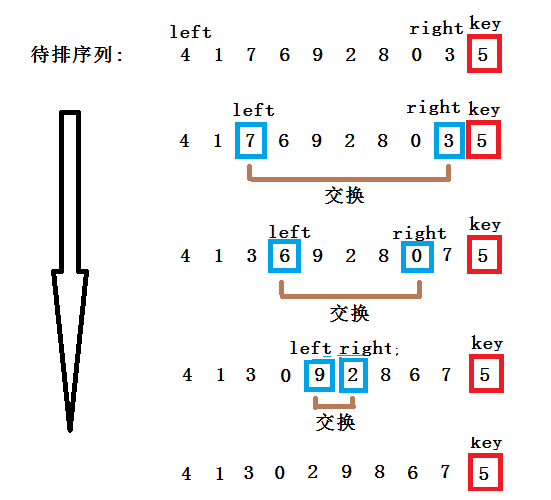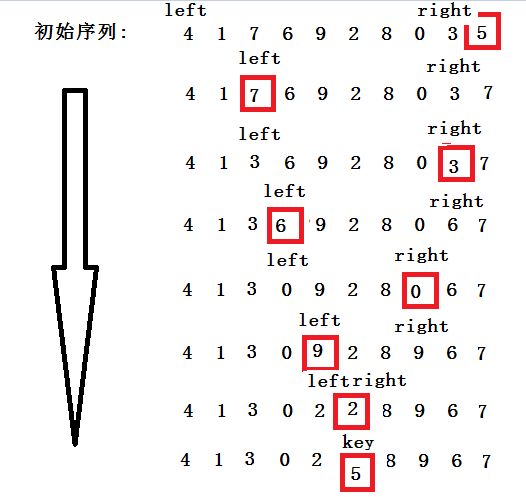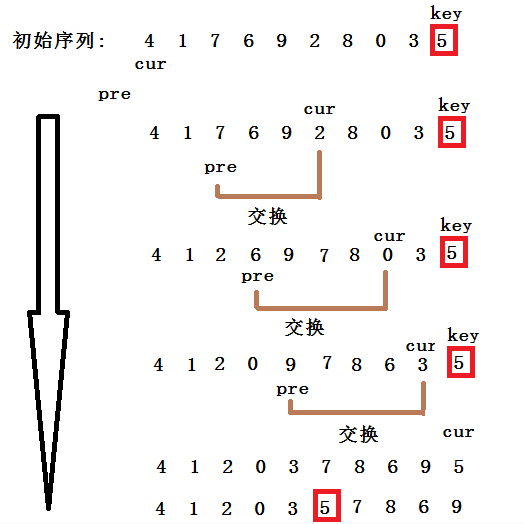# 快速排序(三种算法实现和非递归实现)

void QuickSort(int* array,int left,int right)
{
assert(array);
if(left >= right)//表示已经完成一个组
{
return;
}
int index = PartSort(array,left,right);//枢轴的位置
QuickSort(array,left,index - 1);
QuickSort(array,index + 1,right);
}


PartSort()函数是进行一次快排的算法。

### 左右指针法

1. 选取一个关键字(key)作为枢轴，一般取整组记录的第一个数/最后一个，这里采用选取序列最后一个数为枢轴。
2. 设置两个变量left = 0;right = N - 1;
3. 从left一直向后走，直到找到一个大于key的值，right从后至前，直至找到一个小于key的值，然后交换这两个数。
4. 重复第三步，一直往后找，直到left和right相遇，这时将key放置left的位置即可。int PartSort(int* array,int left,int right)
{
int& key = array[right];
while(left < right)
{
while(left < right && array[left] <= key)
{
++left;
}
while(left < right && array[right] >= key)
{
--right;
}
swap(array[left],array[right]);
}
swap(array[left],key);
return left;
}


while(left < right && array[left] <= key)


key是整段序列最后一个，right是key前一个位置，如果array[right]这个位置的值和key相等，满足array[left] <= key，然后++left,这时候left会走到key的下标处。

###挖坑法

1. 选取一个关键字(key)作为枢轴，一般取整组记录的第一个数/最后一个，这里采用选取序列最后一个数为枢轴，也是初始的坑位。
2. 设置两个变量left = 0;right = N - 1;
3. 从left一直向后走，直到找到一个大于key的值，然后将该数放入坑中，坑位变成了array[left]。
4. right一直向前走，直到找到一个小于key的值，然后将该数放入坑中，坑位变成了array[right]。
5. 重复3和4的步骤，直到left和right相遇，然后将key放入最后一个坑位。int PartSort(int* array,int left,int right)
{
int key = array[right];
while(left < right)
{
while(left < right && array[left] <= key)
{
++left;
}
array[right] = array[left];
while(left < right && array[right] >= key)
{
--right;
}
array[left] = array[right];
}
array[right] = key;
return right;
}


###前后指针法

1. 定义变量cur指向序列的开头，定义变量pre指向cur的前一个位置。
2. 当array[cur] < key时，cur和pre同时往后走，如果array[cur]>key，cur往后走，pre留在大于key的数值前一个位置。
3. 当array[cur]再次 < key时，交换array[cur]和array[pre]。int PartSort(int* array,int left,int right)
{
if(left < right){
int key = array[right];
int cur = left;
int pre = cur - 1;
while(cur < right)
{
while(array[cur] < key && ++pre != cur)//如果找到小于key的值，并且cur和pre之间有距离时则进行交换。注意两个条件的先后位置不能更换，可以参照评论中的解释
{
swap(array[cur],array[pre]);
}
++cur;
}
swap(array[++pre],array[right]);
return pre;
}
return -1;
}


###快速排序的优化

1、三数取中法

2、直接插入

//三数取中
int GetMid(int* array,int left,int right)
{
assert(array);
int mid = left + ((right - left)>>1);
if(array[left] <= array[right])
{
if(array[mid] <  array[left])
return left;
else if(array[mid] > array[right])
return right;
else
return mid;
}
else
{
if(array[mid] < array[right])
return right;
else if(array[mid] > array[left])
return left;
else
return mid;
}

}

//左右指针法
int PartSort1(int* array,int left,int right)
{
assert(array);
int mid = GetMid(array,left,right);
swap(array[mid],array[right]);

int& key = array[right];
while(left < right)
{
while(left < right && array[left] <= key)//因为有可能有相同的值，防止越界，所以加上left < right
++left;
while(left < right && array[right] >= key)
--right;

swap(array[left],array[right]);
}

swap(array[left],key);
return left;
}

//挖坑法
int PartSort2(int* array,int left,int right)
{
assert(array);
int mid = GetMid(array,left,right);
swap(array[mid],array[right]);

int key = array[right];
while(left < right)
{
while(left < right && array[left] <= key)
++left;
array[right] = array[left];

while(left < right && array[right] >= key)
--right;
array[left] = array[right];
}
array[right] = key;
return right;
}

//前后指针法
int PartSort3(int* array,int left,int right)
{
assert(array);
int mid = GetMid(array,left,right);
swap(array[mid],array[right]);
if(left < right){
int key = array[right];
int cur = left;
int pre = left - 1;
while(cur < right)
{
while(array[cur] < key && ++pre != cur)
{
swap(array[cur],array[pre]);
}
++cur;
}
swap(array[++pre],array[right]);
return pre;
}
return -1;
}

void QuickSort(int* array,int left,int right)
{
assert(array);
if(left >= right)
return;

//当序列较短时，采用直接插入
if((right - left) <= 5)
InsertSort(array,right-left+1);

int index = PartSort3(array,left,right);
QuickSort(array,left,index-1);
QuickSort(array,index+1,right);
}

int main()
{
int array[] = {4,1,7,6,9,2,8,0,3,5};
QuickSort(array,0,sizeof(array)/sizeof(array) -1);//因为传的是区间，所以这里要 - 1；
}


## 非递归实现

void QuickSortNotR(int* array,int left,int right)
{
assert(array);
stack<int> s;
s.push(left);
s.push(right);//后入的right，所以要先拿right
while(!s.empty)//栈不为空
{
int right = s.top();
s.pop();
int left = s.top();
s.pop();

int index = PartSort(array,left,right);
if((index - 1) > left)//左子序列
{
s.push(left);
s.push(index - 1);
}
if((index + 1) < right)//右子序列
{
s.push(index + 1);
s.push(right);
}
}
}


01-2309-191万+
07-0226万+
08-1364万+
07-131412
09-1049万+
06-1214万+
07-017万+
10-2084
03-315805
03-206650
09-256507
11-29393
03-231307
07-171万+
02-097100
03-26109
03-176206
07-264万+
09-23156
©️2020 CSDN 皮肤主题: 大白 设计师:CSDN官方博客